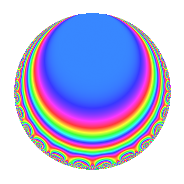# Properties

 Label 4032.2.c.kLevel 4032 Weight 2 Character orbit 4032.c Analytic conductor 32.196 Analytic rank 0 Dimension 4 CM No Inner twists 2

# Related objects

## Newspace parameters

 Level: $$N$$ = $$4032 = 2^{6} \cdot 3^{2} \cdot 7$$ Weight: $$k$$ = $$2$$ Character orbit: $$[\chi]$$ = 4032.c (of order $$2$$ and degree $$1$$)

## Newform invariants

 Self dual: No Analytic conductor: $$32.195682095$$ Analytic rank: $$0$$ Dimension: $$4$$ Coefficient field: $$\Q(\zeta_{12})$$ Coefficient ring: $$\Z[a_1, \ldots, a_{5}]$$ Coefficient ring index: $$2^{5}$$ Sato-Tate group: $\mathrm{SU}(2)[C_{2}]$

## $q$-expansion

Coefficients of the $$q$$-expansion are expressed in terms of a basis $$1,\beta_1,\beta_2,\beta_3$$ for the coefficient ring described below. We also show the integral $$q$$-expansion of the trace form.

 $$f(q)$$ $$=$$ $$q -\beta_{1} q^{5} - q^{7} +O(q^{10})$$ $$q -\beta_{1} q^{5} - q^{7} -2 \beta_{1} q^{11} + ( -2 \beta_{1} + \beta_{3} ) q^{13} -2 q^{17} + \beta_{3} q^{19} + \beta_{2} q^{23} + ( 1 + \beta_{2} ) q^{25} + ( -\beta_{1} - \beta_{3} ) q^{29} -4 q^{31} + \beta_{1} q^{35} + ( -\beta_{1} - \beta_{3} ) q^{37} + 2 q^{41} + 2 \beta_{1} q^{43} + ( -4 - 2 \beta_{2} ) q^{47} + q^{49} + ( -3 \beta_{1} + 3 \beta_{3} ) q^{53} + ( -8 + 2 \beta_{2} ) q^{55} + ( 4 \beta_{1} + \beta_{3} ) q^{59} + ( 5 \beta_{1} + 2 \beta_{3} ) q^{61} + ( -8 + 3 \beta_{2} ) q^{65} + ( 2 \beta_{1} - 2 \beta_{3} ) q^{67} + ( -4 - 2 \beta_{2} ) q^{71} + ( 6 - 2 \beta_{2} ) q^{73} + 2 \beta_{1} q^{77} + ( 4 - 2 \beta_{2} ) q^{79} + ( 3 \beta_{1} + 2 \beta_{3} ) q^{83} + 2 \beta_{1} q^{85} + ( 2 + 4 \beta_{2} ) q^{89} + ( 2 \beta_{1} - \beta_{3} ) q^{91} + \beta_{2} q^{95} + ( 2 - 2 \beta_{2} ) q^{97} +O(q^{100})$$ $$\operatorname{Tr}(f)(q)$$ $$=$$ $$4q - 4q^{7} + O(q^{10})$$ $$4q - 4q^{7} - 8q^{17} + 4q^{25} - 16q^{31} + 8q^{41} - 16q^{47} + 4q^{49} - 32q^{55} - 32q^{65} - 16q^{71} + 24q^{73} + 16q^{79} + 8q^{89} + 8q^{97} + O(q^{100})$$

Basis of coefficient ring:

 $$\beta_{0}$$ $$=$$ $$1$$ $$\beta_{1}$$ $$=$$ $$-\zeta_{12}^{3} + 2 \zeta_{12}^{2} - 1$$ $$\beta_{2}$$ $$=$$ $$-2 \zeta_{12}^{3} + 4 \zeta_{12}$$ $$\beta_{3}$$ $$=$$ $$3 \zeta_{12}^{3} + 2 \zeta_{12}^{2} - 1$$
 $$1$$ $$=$$ $$\beta_0$$ $$\zeta_{12}$$ $$=$$ $$($$$$\beta_{3} + 2 \beta_{2} - \beta_{1}$$$$)/8$$ $$\zeta_{12}^{2}$$ $$=$$ $$($$$$\beta_{3} + 3 \beta_{1} + 4$$$$)/8$$ $$\zeta_{12}^{3}$$ $$=$$ $$($$$$\beta_{3} - \beta_{1}$$$$)/4$$

## Character Values

We give the values of $$\chi$$ on generators for $$\left(\mathbb{Z}/4032\mathbb{Z}\right)^\times$$.

 $$n$$ $$127$$ $$577$$ $$1793$$ $$3781$$ $$\chi(n)$$ $$1$$ $$1$$ $$1$$ $$-1$$

## Embeddings

For each embedding $$\iota_m$$ of the coefficient field, the values $$\iota_m(a_n)$$ are shown below.

For more information on an embedded modular form you can click on its label.

Label $$\iota_m(\nu)$$ $$a_{2}$$ $$a_{3}$$ $$a_{4}$$ $$a_{5}$$ $$a_{6}$$ $$a_{7}$$ $$a_{8}$$ $$a_{9}$$ $$a_{10}$$
2017.1
 −0.866025 − 0.500000i 0.866025 + 0.500000i 0.866025 − 0.500000i −0.866025 + 0.500000i
0 0 0 2.73205i 0 −1.00000 0 0 0
2017.2 0 0 0 0.732051i 0 −1.00000 0 0 0
2017.3 0 0 0 0.732051i 0 −1.00000 0 0 0
2017.4 0 0 0 2.73205i 0 −1.00000 0 0 0
 $$n$$: e.g. 2-40 or 990-1000 Significant digits: Format: Complex embeddings Normalized embeddings Satake parameters Satake angles

## Inner twists

Char. orbit Parity Mult. Self Twist Proved
1.a Even 1 trivial yes
8.b Even 1 yes

## Hecke kernels

This newform can be constructed as the intersection of the kernels of the following linear operators acting on $$S_{2}^{\mathrm{new}}(4032, [\chi])$$:

 $$T_{5}^{4} + 8 T_{5}^{2} + 4$$ $$T_{11}^{4} + 32 T_{11}^{2} + 64$$ $$T_{13}^{4} + 56 T_{13}^{2} + 484$$ $$T_{17} + 2$$ $$T_{23}^{2} - 12$$ $$T_{31} + 4$$